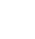# First day of the Month formula

``` =NOW()-DAY(NOW())+1 ```

This simple formula when put in any cell will automatically create the value in that cell as the first day of the current month.

ie if it is 11th January 2019 and you used this formula, it would return:

01/01/2019

Very useful when used with the =EDATE formula